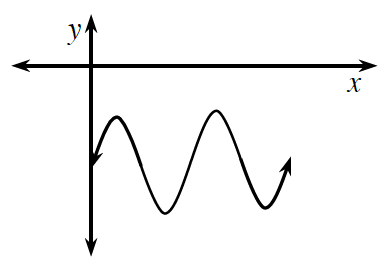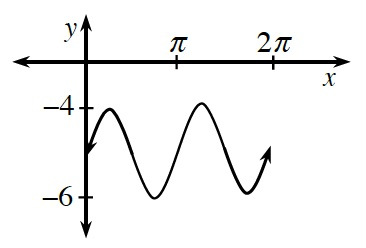### Home > PC3 > Chapter 2 > Lesson 2.3.1 > Problem2-129

2-129.

​Graph two complete cycles of each of the following functions

1. $f(x)=\sin(2x)-5$

Sketch a sine function shifted down $5$ units. Do not scale the axes to begin with.This function has a period of $\frac{2\pi}{2}$, so add a scale to your axes to match this characteristic as well as the amplitude.1. $g(x)=4\cos\left(x+\frac{\pi}{3}\right)$

Follow a process similar to the steps given in part (a).
This time begin by sketching a cosine function that is shifted to the left.